# Python Excel Tutorial: The Definitive Guide

Learn how to read and import Excel files in Python, write data to these spreadsheets, and find the best packages to do this.
Updated Mar 2023  · 30 min read

## Introduction to Excel in Python

Whether you are a student or a working professional, chances are that you have used Excel to work with data and crunch numbers.

In fact, research in 2019 found that roughly 54% of businesses use Excel to perform arithmetic operations, analyze data, create visualizations, and generate reports. You can also perform predictive modeling tasks like regression and clustering using Excel.

However, despite Excel’s undisputed strengths, the tool comes with its own set of drawbacks, making it, at times, inefficient when performing specific tasks involving huge datasets.

One limitation of Excel is its inability to handle large amounts of data. You can run into serious performance issues when trying to perform complex operations on a lot of data entries in Excel, especially if your formulas and macros are not optimized for performance.

Excel can also become very time-consuming if you need to perform repetitive tasks. For instance, if you need to replicate an analysis on multiple Excel files every week, you would have to open them manually and copy-paste the same formulas over and over.

Surveys show that 93% of Excel users find it time-consuming to consolidate spreadsheets and that employees spend approximately 12 hours each month just combining different Excel files.

These drawbacks can be solved by automating Excel workflows with Python. Tasks like spreadsheet consolidation, data cleaning, and predictive modeling can be done in minutes using a simple Python script that writes to an Excel file.

Excel users can also create a scheduler in Python that runs the script automatically at different time intervals, dramatically reducing the amount of human intervention required to perform the same task again and again.

• Use a library called Openpyxl to read and write Excel files using Python
• Create arithmetic operations and Excel formulas in Python
• Manipulate Excel worksheets using Python
• Build visualizations in Python and save them to an Excel file
• Format Excel cell colors and styles using Python

## Introduction to Openpyxl

Openpyxl is a Python library that allows users to read Excel files and write to them.

This framework can help you write functions, format spreadsheets, create reports, and build charts directly in Python without even having to open an Excel application.

Furthermore, Openpyxl allows users to iterate through worksheets and perform the same analysis on multiple sets of data at the same time.

This improves efficiency and allows for the automation of Excel workflows since users only need to perform the analysis on one worksheet and can replicate as many times as required.

## How to Install Openpyxl

To install Openpyxl, simply open your command prompt or Powershell and type the following command:

``\$pip install Openpyxl``

You should see the following message appear indicating that the package is successfully installed: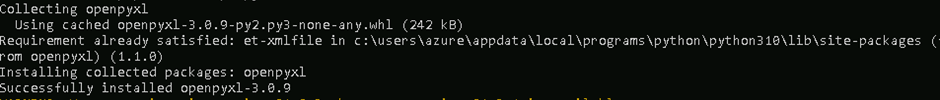## Reading Excel Files in Python with Openpyxl

We will be using Kaggle’s Video Game Sales dataset in this tutorial. This dataset has been preprocessed by our team for the purpose of this tutorial, and you can download the modified version from this link. You can import Excel into Python by following the process below:

``````import openpyxl

Now that the Excel file is loaded as a Python object, you need to tell the library which worksheet to access. There are two ways to do this:

The first method is to simply call the active worksheet, which is the first sheet in the workbook, using the following line of code:

``ws = wb.active``

Alternatively, if you know the name of the worksheet, you can also access it by its name. We will be using the “vgsales” sheet in this section of the tutorial:

``ws = wb['vgsales']``

Let’s now count the number of rows and columns in this worksheet:

``print('Total number of rows: '+str(ws.max_row)+'. And total number of columns: '+str(ws.max_column))``

The above code should render the following output:

``Total number of rows: 16328. And total number of columns: 10``

Now that we know the dimensions of the sheet, let’s move on and learn how to read data from the workbook.

2. ### Reading Data From a Cell

Here is a screenshot of the active sheet that we will be working with in this section: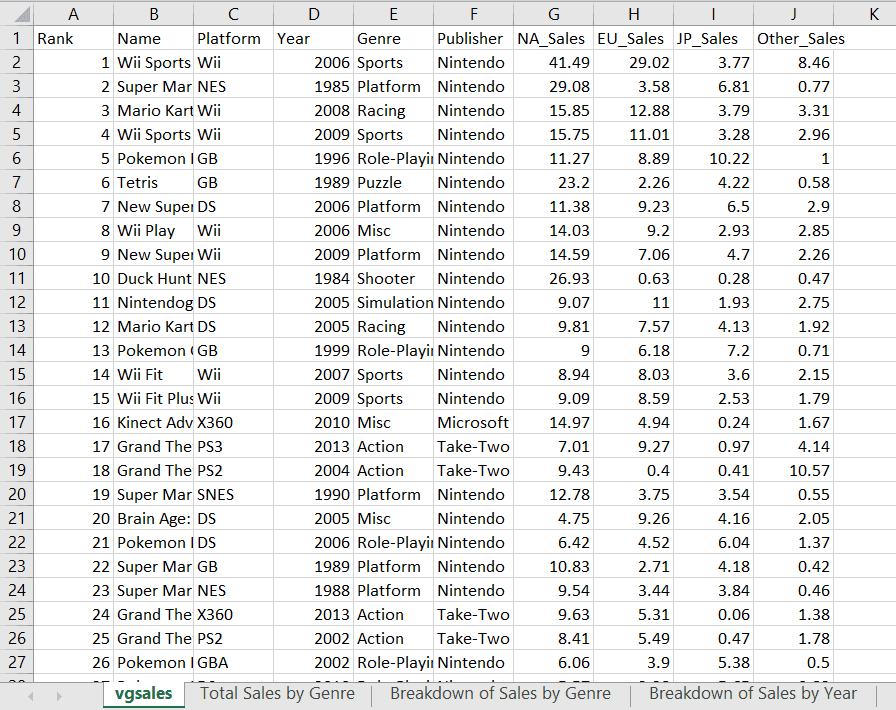To retrieve data from a specific cell with Openpyxl, you can type the cell’s value in like this:

``print('The value in cell A1 is: '+ws['A1'].value)``

You should get the following output:

``The value in cell A1 is: Rank``
3. ### Reading Data From Multiple Cells

Now that we know how to read data from a specific cell, what if we wanted to print all cell values in a certain row of the spreadsheet?

To do this, you can write a simple `for loop` to iterate through all the values in a specific row:

``````values = [ws.cell(row=1,column=i).value for i in range(1,ws.max_column+1)]
print(values)``````

The code above will print out all the values in the first row:

``['Rank', 'Name', 'Platform', 'Year', 'Genre', 'Publisher', 'NA_Sales', 'EU_Sales', 'JP_Sales', 'Other_Sales']``

Next, let’s try printing out multiple rows in a specific column.

We will create a for loop to render the first ten rows in the “Name” column as a list. We should get the names highlighted in the red box below:``````data=[ws.cell(row=i,column=2).value for i in range(2,12)]
print(data)``````

The above code will generate the following output:

``['Wii Sports', 'Super Mario Bros.', 'Mario Kart Wii', 'Wii Sports Resort', 'Pokemon Red/Pokemon Blue', 'Tetris', 'New Super Mario Bros.', 'Wii Play', 'New Super Mario Bros. Wii', 'Duck Hunt']``

Finally, let’s print the first ten rows in a range of columns in the spreadsheet:

``````# reading data from a range of cells (from column 1 to 6)

my_list = list()

for value in ws.iter_rows(
min_row=1, max_row=11, min_col=1, max_col=6,
values_only=True):
my_list.append(value)

for ele1,ele2,ele3,ele4,ele5,ele6 in my_list:
(print ("{:<8}{:<35}{:<10}
{:<10}{:<15}{:<15}".format(ele1,ele2,ele3,ele4,ele5,ele6)))``````

The first ten rows of data in the first six columns should be displayed after you run the above code: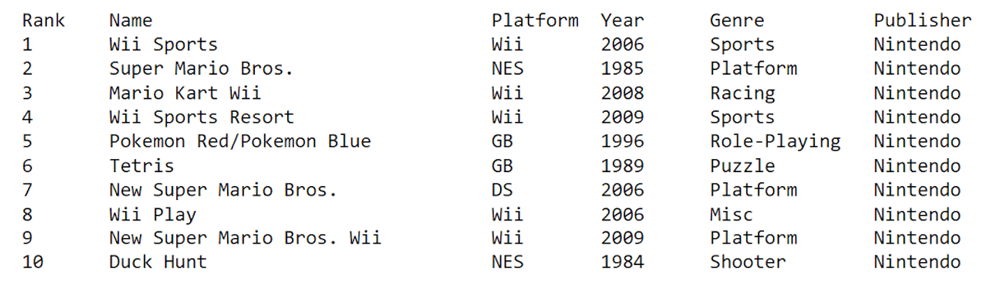## Writing to Excel Files with Openpyxl

Now that we know how to access and read data from Excel files, let’s learn how to write to them using Openpyxl.

1. ### Writing to a Cell

There are two ways you can write to a file with Openpyxl.

First, you can access the cell directly using its key:

``ws['K1'] = 'Sum of Sales'``

An alternative is to specify the row and column position of the cell that you’d like to write to:

``ws.cell(row=1, column=11, value = 'Sum of Sales')``

Every time you write to an Excel file with Openpyxl, you need to save your changes with the following line of code or they will not be reflected in the worksheet:

``wb.save('videogamesales.xlsx')``

If your workbook is open when you try to save it, you will run into the following permission error: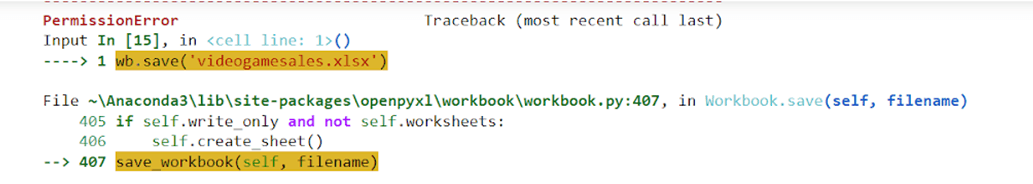Make sure to close the Excel file before saving your changes. You can then open it again to make sure that the change is reflected in your worksheet: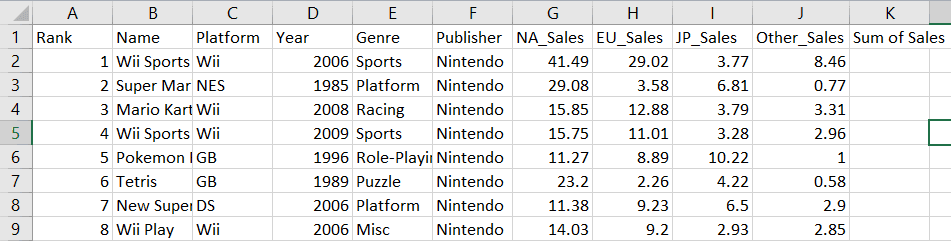Notice that a new column called “Sum of Sales” has been created in cell K1.

2. ### Creating a New Column

Let’s now add the sum of sales in every region and write it to column K.

We will do this for the sales data in the first row:

``````row_position = 2
col_position = 7

total_sales = ((ws.cell(row=row_position, column=col_position).value)+
(ws.cell(row=row_position, column=col_position+1).value)+
(ws.cell(row=row_position, column=col_position+2).value)+
(ws.cell(row=row_position, column=col_position+3).value))

ws.cell(row=2,column=11).value=total_sales
wb.save('videogamesales.xlsx')``````

Notice that the total sales have been calculated in cell K2 for the first game in the worksheet: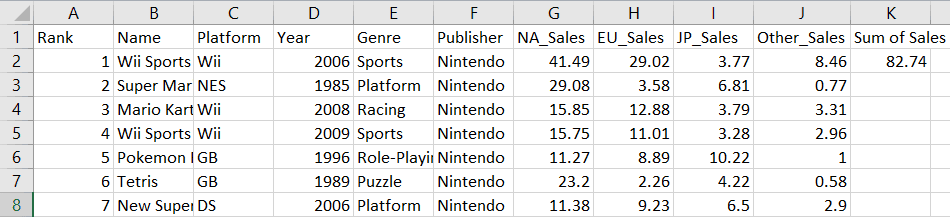Similarly, let’s create a for loop to sum the sales values in every row:

``````row_position = 1

for i in range(1, ws.max_row):

row_position += 1
NA_Sales = ws.cell(row=row_position, column=7).value
EU_Sales = ws.cell(row=row_position, column=8).value
JP_Sales = ws.cell(row=row_position, column=9).value
Other_Sales = ws.cell(row=row_position, column=10).value

total_sales = (NA_Sales + EU_Sales + JP_Sales + Other_Sales)
ws.cell(row=row_position, column=11).value = total_sales

wb.save("videogamesales.xlsx")``````

Your Excel file should now have a new column reflecting the total sales of video games in all regions: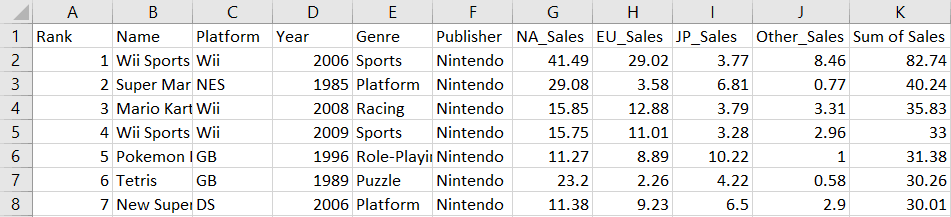3. ### Appending New Rows

To append a new row to the workbook, simply create a tuple with the values you’d like to include and write it to the sheet:

``````new_row = (1,'The Legend of Zelda',1986,'Action','Nintendo',3.74,0.93,1.69,0.14,6.51,6.5)

ws.append(new_row)

wb.save('videogamesales.xlsx')``````

You can confirm that this data has been appended by printing the last row in the workbook:

``````values = [ws.cell(row=ws.max_row,column=i).value for i in range(1,ws.max_column+1)]
print(values)``````

The following output will be generated:

``[1, 'The Legend of Zelda', 1986, 'Action', 'Nintendo', 3.74, 0.93, 1.69, 0.14, 6.51, 6.5]``
4. ### Deleting Rows

To delete the new row we just created, you can run the following line of code:

``````ws.delete_rows(ws.max_row, 1) # row number, number of rows to delete

wb.save('videogamesales.xlsx')``````

The first argument in the delete_rows() function is the row number you want to delete. The second argument indicates the number of rows that should be deleted.

## Creating Excel Formulas with Openpyxl

You can use Openpyxl to write formulas exactly like you would in Excel. Here are some examples of basic functions you can create using Openpyxl:

1. ### AVERAGE

Let’s create a new column called “Average Sales” to calculate the average total video game sales in all markets:

``````ws['P1'] = 'Average Sales'
ws['P2'] = '= AVERAGE(K2:K16220)'

wb.save('videogamesales.xlsx')``````

The average sales across all markets is approximately 0.19. This will be printed in cell P2 of your worksheet.

2. ### COUNTA

The “COUNTA” function in Excel counts cells that are populated within a specific range. Let’s use it to find the number of records between E2 and E16220:

``````ws['Q1'] = "Number of Populated Cells"
ws['Q2'] = '=COUNTA(E2:E16220)'

wb.save('videogamesales.xlsx')``````

There are 16,219 records in this range that have information in them.

3. ### COUNTIF

COUNTIF is a commonly used Excel function that counts the number of cells that meet a specific condition. Let’s use it to count the number of games in this dataset with the “Sports” genre:

``````ws['R1'] = 'Number of Rows with Sports Genre'
ws['R2'] = '=COUNTIF(E2:E16220, "Sports")'

wb.save('videogamesales.xlsx')``````

There are 2,296 sports games in the dataset.

4. ### SUMIF

Now, let’s find the total “Sum of Sales” generated by sports games using the SUMIF function:

``````ws['S1'] = 'Total Sports Sales'
ws['S2'] = '=SUMIF(E2:E16220, "Sports",K2:K16220)'

wb.save('videogamesales.xlsx')``````

The total number of sales generated by sports games is 454.

5. ### CEILING

The CEILING function in Excel rounds a number up to the nearest specified multiple. Let’s round up the total amount of sales generated by sports games using this function:

``````ws['T1'] = 'Rounded Sum of Sports Sales'
ws['T2'] = '=CEILING(S2,25)'

wb.save('videogamesales.xlsx')``````

We have rounded the total sales generated by sports games to the nearest multiple of 25, which returns a result of 475.

The code snippets above should generate the following output in your Excel sheet (from cells P1 to T2):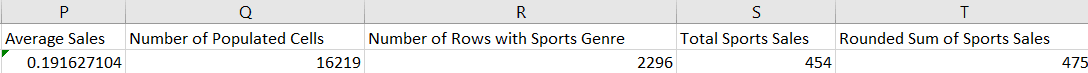You can refer to our Excel Basics Cheat Sheet to learn more about Excel formulas, operators, math functions, and conditional computation.

## Working with Sheets in Openpyxl

Now that we know how to access worksheets and write to them, let’s learn how we can manipulate, remove, and duplicate them using Openpyxl.

1. ### Changing Sheet Names

First, let’s print the name of the active sheet we are currently working with using Openpyxl’s title attribute:

``print(ws.title)``

The following output will be rendered:

``vgsales``

Now, let’s rename this worksheet using the following lines of code:

``````ws.title ='Video Game Sales Data'

wb.save('videogamesales.xlsx')``````

The name of your active sheet should now be changed to “Video Game Sales Data.”

2. ### Creating a New Worksheet

Run the following line of code to list all the worksheets in the workbook:

``print(wb.sheetnames)``

You will see an array listing the names of all the worksheets in the file:

``['Video Game Sales Data', 'Total Sales by Genre', 'Breakdown of Sales by Genre', 'Breakdown of Sales by Year']``

Let’s now create a new empty worksheet:

``````wb.create_sheet('Empty Sheet') # create an empty sheet
print(wb.sheetnames) # print sheet names again

wb.save('videogamesales.xlsx')``````

Notice that a new sheet called “Empty Sheet” has now been created:

``['Video Game Sales Data', 'Total Sales by Genre', 'Breakdown of Sales by Genre', 'Breakdown of Sales by Year', ‘Empty Sheet’]``
3. ### Deleting a Worksheet

To delete a worksheet using Openpyxl, simply use the remove attribute and print all the sheet names again to confirm that the sheet has been deleted:

``````wb.remove(wb['Empty Sheet'])
print(wb.sheetnames)

wb.save('videogamesales.xlsx')``````

Notice that the “Empty Sheet” worksheet is no longer available:

``['Video Game Sales Data', 'Total Sales by Genre', 'Breakdown of Sales by Genre', 'Breakdown of Sales by Year']``
4. ### Duplicating a Worksheet

Finally, run this line of code to create a copy of an existing worksheet:

``````wb.copy_worksheet(wb['Video Game Sales Data'])
wb.save('vgsales_2.xlsx')``````

Printing all the sheet names again, we get the following output:

``['Video Game Sales Data', 'Total Sales by Genre', 'Breakdown of Sales by Genre', 'Breakdown of Sales by Year', 'Video Game Sales Data Copy']``

## Adding Charts to an Excel File with Openpyxl

Excel is often seen as the go-to tool for creating visualizations and summarizing datasets. In this section, we will learn to build charts in Excel directly from Python using Openpyxl.

1. ### Bar Chart

Let’s first create a simple bar chart displaying the total sales of video games by genre. We will be using the “Total Sales by Genre” worksheet to do this: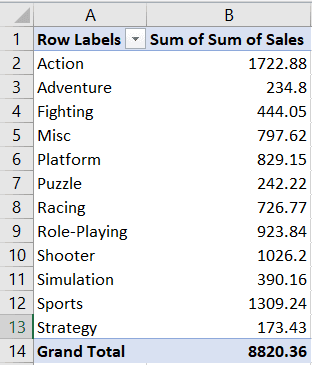This worksheet contains a pivot table in which the sum of sales has been aggregated by genre, as seen in the screenshot above.

Let’s access this worksheet before we start creating the bar chart:

``ws = wb['Total Sales by Genre'] # access the required worksheet``

Now, we need to tell Openpyxl the values and categories that we’d like to plot.

#### Values:

The values include the “Sum of Sales” data that we want to plot. We need to tell Openpyxl where to find this data in the Excel file by including the range in which your values begin and end.

Four parameters in Openpyxl allow you to specify where your values are located:

• Min_column: The minimum column containing data
• Max_column: The maximum column containing data
• Min_row: The minimum row containing data
• Max_row: The maximum row containing data

Here is an image showcasing how you can define these parameters: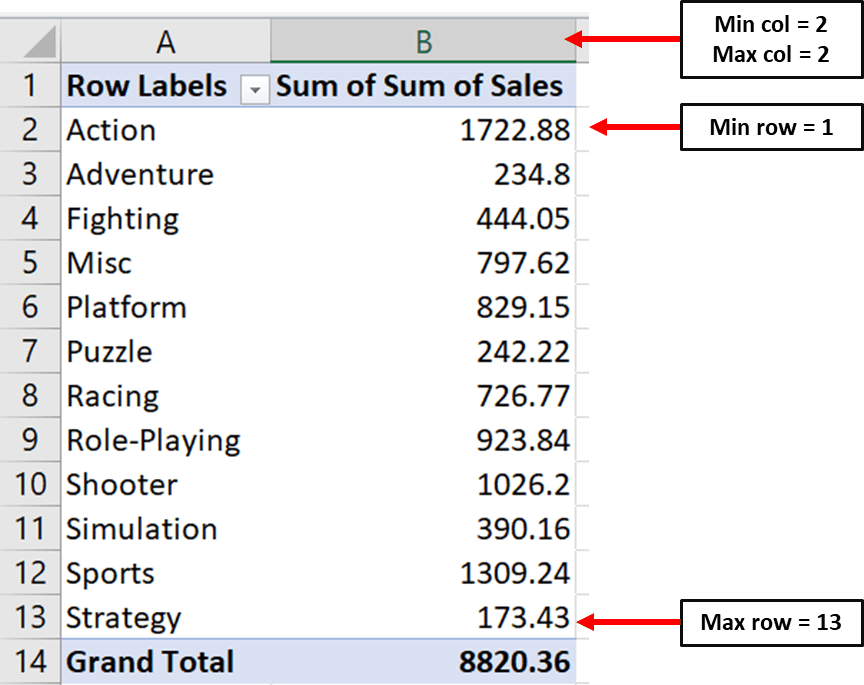Notice that the minimum row is the first row and not the second one. This is because Openpyxl starts counting from the row that has a numeric value in it.

``````# Values for plotting

from openpyxl.chart import Reference

values = Reference(ws,         # worksheet object
min_col=2,  # minimum column where your values begin
max_col=2,  # maximum column where your values end
min_row=1,  # minimum row you’d like to plot from
max_row=13) # maximum row you’d like to plot from``````

#### Categories

Now, we need to define the same parameters for the categories in our bar chart: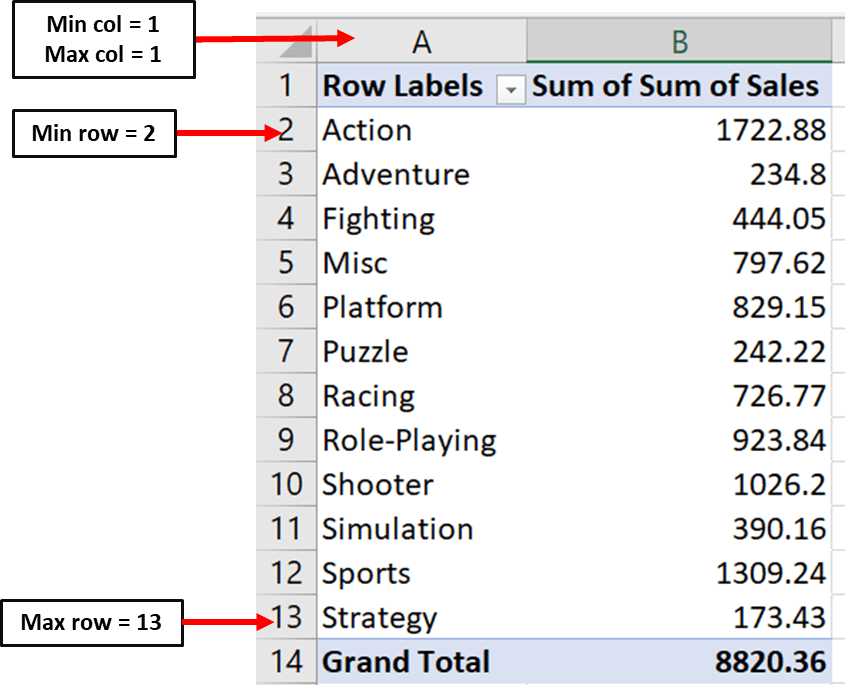Here is the code you can use to set parameters for the chart’s categories:

``````cats = Reference(ws,
min_col=1,
max_col=1,
min_row=2,
max_row=13)``````

#### Creating the Bar Chart

Now, we can create the bar chart object and include our values and categories using the following lines of code:

``````from openpyxl.chart import BarChart

chart = BarChart()
chart.set_categories(cats)``````

#### Set Chart Titles

Finally, you can set the chart titles and tell Openpyxl where you’d like to create it in the Excel sheet:

``````# set the title of the chart
chart.title = "Total Sales"

# set the title of the x-axis
chart.x_axis.title = "Genre"

# set the title of the y-axis
chart.y_axis.title = "Total Sales by Genre"

# the top-left corner of the chart
# is anchored to cell F2 .

# save the file
wb.save("videogamesales.xlsx")``````

You can then open the Excel file and navigate to the “Total Sales by Genre” worksheet. You should notice a chart that looks like this appear: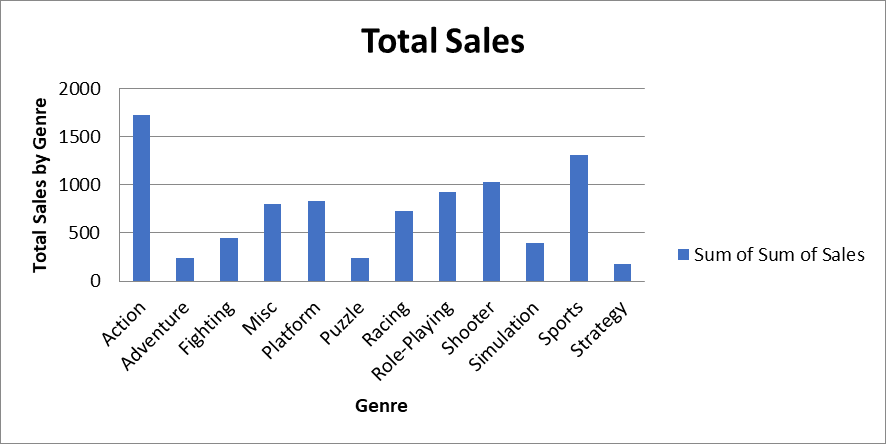2. ### Grouped Bar Chart

Now, let’s create a grouped bar chart displaying total sales by genre and region. You can find the data for this chart in the “Breakdown of Sales by Genre” worksheet: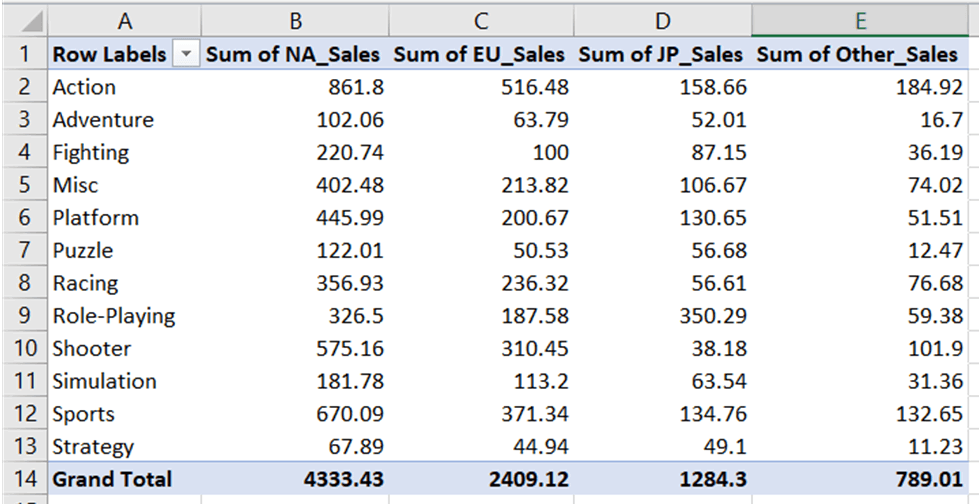Similar to how we created the bar chart, we need to define the range for values and categories:We can now access the worksheet and write this down in code:

``````### Creating a Grouped Bar Chart with Openpyxl

ws = wb['Breakdown of Sales by Genre'] # access worksheet

# Data for plotting

values = Reference(ws,
min_col=2,
max_col=5,
min_row=1,
max_row=13)

cats = Reference(ws, min_col=1,
max_col=1,
min_row=2,
max_row=13)``````

We can now create the bar chart object, include the values and categories in it, and set the title parameters exactly like we did previously:

``````# Create object of BarChart class

chart = BarChart()
chart.set_categories(cats)

# set the title of the chart
chart.title = "Sales Breakdown"

# set the title of the x-axis
chart.x_axis.title = "Genre"

# set the title of the y-axis
chart.y_axis.title = "Breakdown of Sales by Genre"

# the top-left corner of the chart is anchored to cell H2.

# save the file
wb.save("videogamesales.xlsx")``````

Once you open the worksheet, a grouped bar chart that looks like this should appear: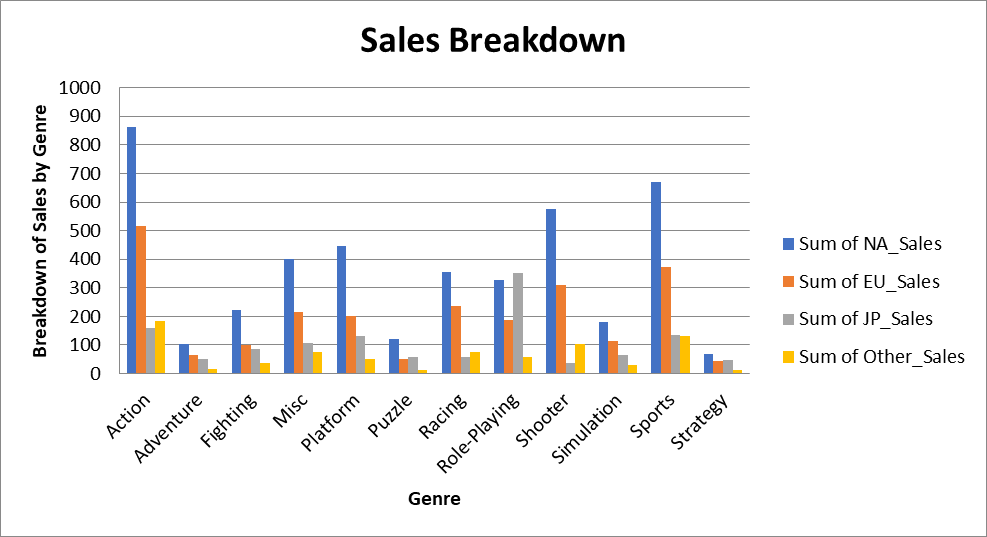3. ### Stacked Line Chart

Finally, we will create a stacked line chart using data in the tab “Breakdown of Sales by Year.” This worksheet contains video game sales data broken down by year and region: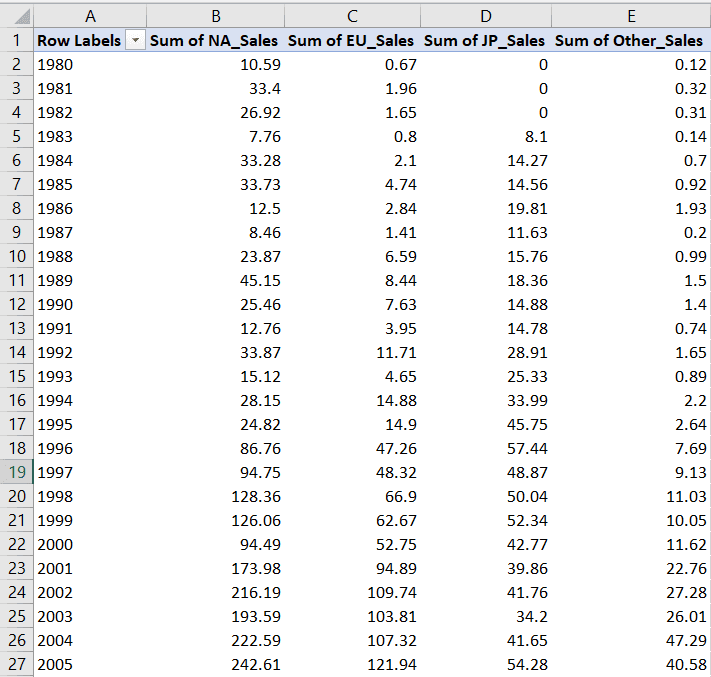Let’s define the range for this chart’s values and categories:We can now write these minimum and maximum values in code:

``````# Data for plotting

values = Reference(ws,
min_col=2,
max_col=6,
min_row=1,
max_row=40)

cats = Reference(ws, min_col=1, max_col=1, min_row=2, max_row=40)``````

Finally, let’s create the line chart object and set the graph’s title, x-axis, and y-axis:

``````# Create object of LineChart class

from openpyxl.chart import LineChart

chart = LineChart()
chart.set_categories(cats)

# set the title of the chart
chart.title = "Total Sales"

# set the title of the x-axis
chart.x_axis.title = "Year"

# set the title of the y-axis
chart.y_axis.title = "Total Sales by Year"

# the top-left corner of the chart is anchored to cell H2

# save the file
wb.save("videogamesales.xlsx")``````

A stacked line chart that looks like this should appear on your worksheet: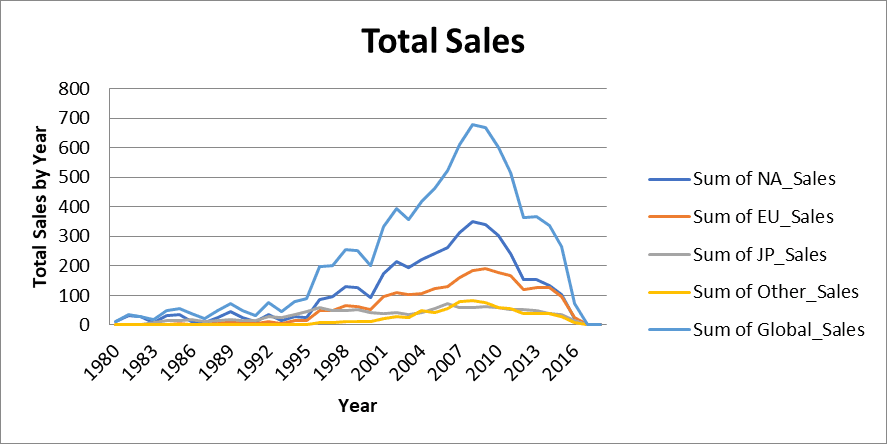## Formatting Cells Using Openpyxl

Openpyxl allows users to style cells in Excel workbooks. You can make your spreadsheet look prettier by changing font sizes, background colors, and cell borders directly in Python.

Here are some ways to customize your Python Excel spreadsheet using Openpyxl:

1. ### Changing Font Sizes and Styles

Let’s increase the font size in cell A1 and bold the text using the following lines of code:

``````from openpyxl.styles import Font

ws = wb['Video Game Sales Data']
ws['A1'].font = Font(bold=True, size=12)

wb.save('videogamesales.xlsx')``````

Notice that the text in cell A1 is now slightly larger and bolded: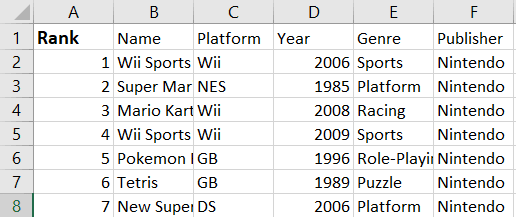Now, what if we wanted to change the font size and style for all the column headers in the first row?

To do this, we can use the same code and simply create a for loop to iterate through all the columns in the first row:

``````for cell in ws["1:1"]:
cell.font = Font(bold=True, size=12)

wb.save('videogamesales.xlsx')``````

When we iterate through [“1:1”], we are telling Openpyxl the start and end rows to loop through. If we wanted to loop through the first ten rows, for instance, then we’d specify [“1:10”] instead.

You can open the Excel sheet to check if the changes have been reflected: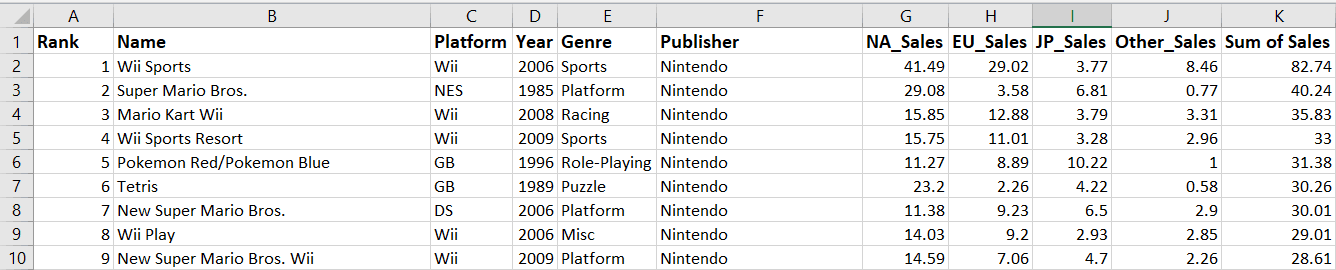2. ### Changing Font Color

You can change font colors in Openpyxl using hex codes:

``````from openpyxl.styles import colors

ws['A1'].font = Font(color = 'FF0000',bold=True, size=12) ## red
ws['A2'].font = Font(color = '0000FF') ## blue

wb.save('videogamesales.xlsx')``````

After saving the workbook and opening it again, the font colors in cells A1 and A2 should have changed: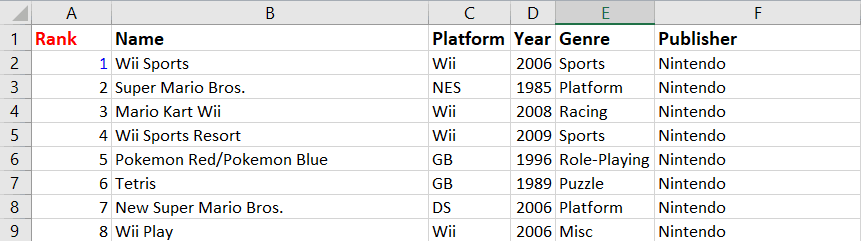3. ### Changing Cell Background Color

To change the background color of a cell, you can use Openpyxl’s PatternFill module:

``````## changing background color of a cell

from openpyxl.styles import PatternFill

ws["A1"].fill = PatternFill('solid', start_color="38e3ff") # light blue background color

wb.save('videogamesales.xlsx')``````

The following change should be reflected in your worksheet: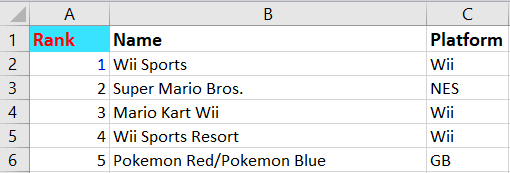To add a cell border using Openpyxl, run the following lines of code:

``````## cell borders

from openpyxl.styles import Border, Side

my_border = Side(border_style="thin", color="000000")

ws["A1"].border = Border(
top=my_border, left=my_border, right=my_border, bottom=my_border
)

wb.save("videogamesales.xlsx")``````

You should see a border that looks like this appear across cell A1: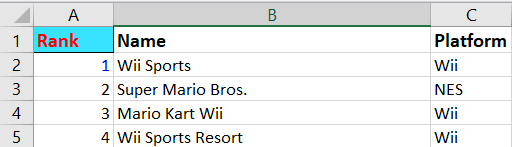5. ### Conditional Formatting

Conditional formatting is the process of highlighting specific values in an Excel file based on a set of conditions. It allows users to visualize data more easily and better understand the values in their worksheets.

Let’s use Openpyxl to highlight in green all the video game sales values that are greater than or equal to 8:

``````from openpyxl.formatting.rule import CellIsRule

fill = PatternFill(
start_color='90EE90',
end_color='90EE90',fill_type='solid') # specify background color

'G2:K16594', CellIsRule(operator='greaterThan', formula=, fill=fill)) # include formatting rule

wb.save('videogamesales.xlsx')``````

In the first block of code, we specify the background color of the cells we’d like to format. In this case, the color is light green.

Then, we create a conditional formatting rule stating that any value greater than 8 should be highlighted in the fill color we specified. We also state the range of cells in which we’d like to apply this condition.

After running the above code, all the sales values above 8 should be highlighted like this: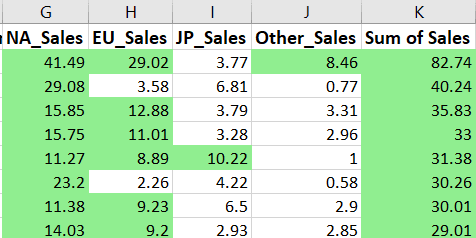## Working with Excel in Python: Next Steps

We have covered a lot of ground in this tutorial, starting from the basics of using the Openpyxl library to performing more advanced operations like creating charts and formatting spreadsheets in Python.

On their own, Python and Excel are powerful data manipulation tools used to build predictive models, produce analytical reports, and perform mathematical calculations.

The biggest advantage of Excel is that it is used by almost everybody. From non-technical stakeholders to entry-level individuals, employees of all levels understand reports presented in an Excel spreadsheet.

Python, on the other hand, is used to analyze and build models on large amounts of data. It can help teams automate laborious tasks and improve organizational efficiency.

When Excel and Python are used together, it can shave hours off a company’s workflows while still sticking to an interface that everyone in the organization is familiar with.

Now that you understand how Openpyxl can be used to work with Excel sheets, here are some ways you can take this newfound knowledge further and use it to add value to your existing workflows:

1. ### Practice on larger datasets

The dataset we used above has only around 16,000 rows, while Openpyxl can handle much larger amounts using the library’s optimized modes. If your goal is to perform operations on large Excel workbooks quickly, you can practice using Openpyxl in its optimized read and write modes.
2. ### Take an online course

While we have covered the fundamentals of working with Excel in Python, there are many concepts that were outside the scope of this course - including working with multiple Excel sheets, creating pivot tables, and summarizing large amounts of data.

We suggest taking Datacamp’s Python for Spreadsheet Users course to bridge some of these gaps in your knowledge.
3. ### Learn to automate Excel workflows in Python

As mentioned earlier in this section, the biggest advantage of using libraries like Openpyxl is the ability to work with multiple workbooks at once and schedule workflows so they don’t have to be repeated many times.

You can try to create a simple function that iterates through multiple workbooks at once and carries out some of the operations covered in this tutorial.
4. ### Learn About Different Libraries

While Openpyxl is one option to manipulate Excel files with Python, there are alternatives like the Pandas library which can help you process Excel content faster.

If cell formatting or working directly with Excel formulas isn’t a requirement for you, Pandas might actually be easier to learn because it has better documentation and community support.

You can take our Data Manipulation with Pandas course to start learning about the library today.

Beginner
4 hr
22.1K
Use your knowledge of common spreadsheet functions and techniques to explore Python!
See Details

### Pandas Joins for Spreadsheet Users

Beginner
4 hr
3.1K
Learn how to effectively and efficiently join datasets in tabular format using the Python Pandas library.

### Introduction to Importing Data in Python

Beginner
3 hr
254.9K
Learn to import data into Python from various sources, such as Excel, SQL, SAS and right from the web.
See More
Related

### Building Your Data Science Portfolio with DataCamp Workspace (Part 1)

Learn how to build a comprehensive data science portfolio by exploring examples different examples, mastering tips to make your work stand out, and utilizing the DataCamp Workspace effectively to showcase your results.9 min

### Pandas 2.0: What’s New and Top Tips

Dive into pandas 2.0, the latest update of the essential data analysis library, with new features like PyArrow integration, nullable data types, and non-nanosecond datetime resolution for better performance and efficiency.### Building a Safer Internet with Data Science

Learn the key drivers of a data strategy that helps ensure online safety and consumer protection with Richard Davis, the Chief Data Officer at Ofcom, the UK’s government-approved regulatory and competition authority.

### Conda Cheat Sheet

In this cheat sheet, learn all about the basics of working with Conda. From managing and installing packages, to working with channels & environments, learn the fundamentals of the conda package management tool suite.

### Data Manipulation in Excel Cheat Sheet

Discover how to manipulate data in Excel using this convenient cheat sheet!

### Matplotlib time series line plot

This tutorial explores how to create and customize time series line plots in matplotlib.
See MoreSee More